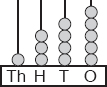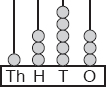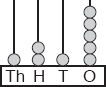# User ForumSubject :IMO    Class : Class 3

Which of the given abacus shows the number less than 1345?

ABCDa is the correct answer

Class : Class 3
D

## Ans 2:

Class : Class 4
read the question . We have to find lesser than 1345 .Option A is the same number given .Option B is greater than the number given D is answer

## Ans 3:

Class : Class 2
A IS CORRECT ANSWER

Class : Class 4
The answer is B.

## Ans 5:

Class : Class 8
The correct answer is D

Class : Class 4

Class : Class 4

Class : Class 3
you idiot

Class : Class 4
The answer is D.

Class : Class 4

Class : Class 9
A